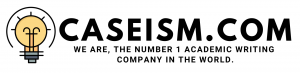# Kohler Company (A) Case Solution & Answer

## Kohler Company (A) Case Solution

### Multiple Valuation Technique:

The multiple Valuation technique is used to calculate the enterprise value per share. Kohler Company (A) per share price can be calculated by using different multiples in comparison of competitors, that is sales multiples, earnings before interest and tax (EBIT) multiple, earnings before interest, tax, amortization and depreciation (EBITDA) multiple or cash flow multiple. Sales, EBITDA, EBIT and Cash flow multiple, per share price is calculated by multiplying the value of sales, EBITDA, EBIT and Cash Flow as the case may be, of Kohler Company (A) with the average respective multiple ratio of the competitor. The enterprise value is calculated by using the sales multiples and is \$328,332 per share.The enterprise value per share in earnings before interest, tax amortization and depreciation (EBITDA) multiple are \$215,368. Per share enterprise value using the earnings before interest and tax (EBIT) is \$186,983. Using the cash flow multiples per share enterprise value of Kohler Company is \$241,925. All these calculations-are shown in exhibit 1.

### Weighted Average Cost of Capital (WACC):

Weighted average cost of capital (WACC) is the average rate of return which is used to discount the cash flow to calculate the present value of the cash flows. Weighted average cost of capital is the minimum required rate of return of the company above which the investment pays off. The formula for calculating weighted average cost of capital is given below.

Where,

We = Percentage of equity in total enterprise value

Ke= Cost of Equity

Wd = Percentage of equity in total enterprise value

Kd = Cost of Debts

In order to calculate weighted average cost of capital, cost of equity and cost of debt of Kohler Company (A) is to be calculated first.

### Cost of Equity (Ke):

Cost of equity of the Kohler Company (A) is calculated by using the Capital Assets Pricing Model (CAPM). Capital asset pricing model (CAPM) owns a formula as under:

Since, beta for the company is not available therefore Proxy beta has been used to calculate the cost of equity stock. Proxy beta can be calculated by using the beta of the competitors of similar nature in the same market.Asset beta of the competitor is calculated by using the formula given below,

Assets beta of all the competitors of Kohler Company (A) is calculated by using the above formula, which is 1.21 for American Standard, 0.81 for the American Wood mark, 1.42 for Masco, 0.80 for Brigs & Stratton and 1.39 for Cummins Engine using a tax rate of 1.52 percent.As shown in exhibit 2.Average asset beta of the industry is used for the purpose of calculation of the proxy equity beta for the Kohler Company (A). Average asset beta of the competitors in the industry is 1.19. Now using this average beta, equity beta would be calculated by using this average beta by using the capital structure of the Kohler Company (A). Therefore, the proxy equity beta of the Kohler Company (A) is 1.4.

Capital asset pricing model (CAPM) is applicable under few assumptions, which are; risk free rate of return is assumed to be 6 percent and the market risk premium is 7 percent as mentioned in the case. The cost of equity of the Kohler Company (A) is 15.77 percent by using the capital asset pricing model (CAPM). As shown in exhibit 3.Other limitations of the capital asset pricing model (CAPM) are; investor need to have a diversified portfolio, there is risk free rate of return for the underlying investment,market is assumed to be perfect and there is a constant premium goes through out the period…………….

This is just a sample partial case solution. Please place the order on the website to order your own originally done case solution.